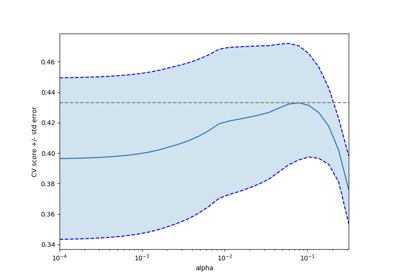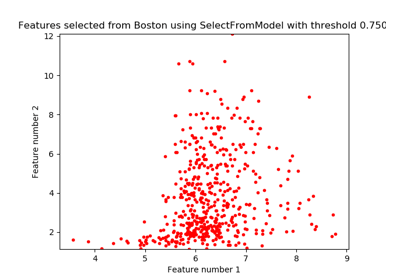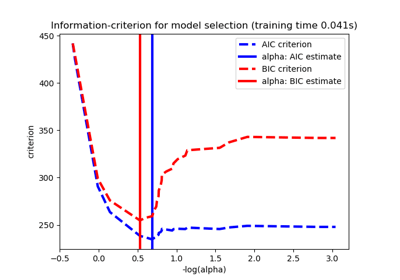/scikit-learn

# 3.2.4.1.3. sklearn.linear_model.LassoCV

class sklearn.linear_model.LassoCV(eps=0.001, n_alphas=100, alphas=None, fit_intercept=True, normalize=False, precompute=’auto’, max_iter=1000, tol=0.0001, copy_X=True, cv=’warn’, verbose=False, n_jobs=None, positive=False, random_state=None, selection=’cyclic’) [source]

Lasso linear model with iterative fitting along a regularization path

The best model is selected by cross-validation.

The optimization objective for Lasso is:

(1 / (2 * n_samples)) * ||y - Xw||^2_2 + alpha * ||w||_1


Read more in the User Guide.

Parameters: eps : float, optional Length of the path. eps=1e-3 means that alpha_min / alpha_max = 1e-3. n_alphas : int, optional Number of alphas along the regularization path alphas : numpy array, optional List of alphas where to compute the models. If None alphas are set automatically fit_intercept : boolean, default True whether to calculate the intercept for this model. If set to false, no intercept will be used in calculations (e.g. data is expected to be already centered). normalize : boolean, optional, default False This parameter is ignored when fit_intercept is set to False. If True, the regressors X will be normalized before regression by subtracting the mean and dividing by the l2-norm. If you wish to standardize, please use sklearn.preprocessing.StandardScaler before calling fit on an estimator with normalize=False. precompute : True | False | ‘auto’ | array-like Whether to use a precomputed Gram matrix to speed up calculations. If set to 'auto' let us decide. The Gram matrix can also be passed as argument. max_iter : int, optional The maximum number of iterations tol : float, optional The tolerance for the optimization: if the updates are smaller than tol, the optimization code checks the dual gap for optimality and continues until it is smaller than tol. copy_X : boolean, optional, default True If True, X will be copied; else, it may be overwritten. cv : int, cross-validation generator or an iterable, optional Determines the cross-validation splitting strategy. Possible inputs for cv are: None, to use the default 3-fold cross-validation, integer, to specify the number of folds. An object to be used as a cross-validation generator. An iterable yielding train/test splits. For integer/None inputs, KFold is used. Refer User Guide for the various cross-validation strategies that can be used here. Changed in version 0.20: cv default value if None will change from 3-fold to 5-fold in v0.22. verbose : bool or integer Amount of verbosity. n_jobs : int or None, optional (default=None) Number of CPUs to use during the cross validation. None means 1 unless in a joblib.parallel_backend context. -1 means using all processors. See Glossary for more details. positive : bool, optional If positive, restrict regression coefficients to be positive random_state : int, RandomState instance or None, optional, default None The seed of the pseudo random number generator that selects a random feature to update. If int, random_state is the seed used by the random number generator; If RandomState instance, random_state is the random number generator; If None, the random number generator is the RandomState instance used by np.random. Used when selection == ‘random’. selection : str, default ‘cyclic’ If set to ‘random’, a random coefficient is updated every iteration rather than looping over features sequentially by default. This (setting to ‘random’) often leads to significantly faster convergence especially when tol is higher than 1e-4. alpha_ : float The amount of penalization chosen by cross validation coef_ : array, shape (n_features,) | (n_targets, n_features) parameter vector (w in the cost function formula) intercept_ : float | array, shape (n_targets,) independent term in decision function. mse_path_ : array, shape (n_alphas, n_folds) mean square error for the test set on each fold, varying alpha alphas_ : numpy array, shape (n_alphas,) The grid of alphas used for fitting dual_gap_ : ndarray, shape () The dual gap at the end of the optimization for the optimal alpha (alpha_). n_iter_ : int number of iterations run by the coordinate descent solver to reach the specified tolerance for the optimal alpha.

#### Notes

For an example, see examples/linear_model/plot_lasso_model_selection.py.

To avoid unnecessary memory duplication the X argument of the fit method should be directly passed as a Fortran-contiguous numpy array.

#### Examples

>>> from sklearn.linear_model import LassoCV
>>> from sklearn.datasets import make_regression
>>> X, y = make_regression(noise=4, random_state=0)
>>> reg = LassoCV(cv=5, random_state=0).fit(X, y)
>>> reg.score(X, y)
0.9993...
>>> reg.predict(X[:1,])
array([-78.4951...])


#### Methods

 fit(X, y) Fit linear model with coordinate descent get_params([deep]) Get parameters for this estimator. path(X, y[, eps, n_alphas, alphas, …]) Compute Lasso path with coordinate descent predict(X) Predict using the linear model score(X, y[, sample_weight]) Returns the coefficient of determination R^2 of the prediction. set_params(**params) Set the parameters of this estimator.
__init__(eps=0.001, n_alphas=100, alphas=None, fit_intercept=True, normalize=False, precompute=’auto’, max_iter=1000, tol=0.0001, copy_X=True, cv=’warn’, verbose=False, n_jobs=None, positive=False, random_state=None, selection=’cyclic’) [source]
fit(X, y) [source]

Fit linear model with coordinate descent

Fit is on grid of alphas and best alpha estimated by cross-validation.

Parameters: X : {array-like}, shape (n_samples, n_features) Training data. Pass directly as Fortran-contiguous data to avoid unnecessary memory duplication. If y is mono-output, X can be sparse. y : array-like, shape (n_samples,) or (n_samples, n_targets) Target values
get_params(deep=True) [source]

Get parameters for this estimator.

Parameters: deep : boolean, optional If True, will return the parameters for this estimator and contained subobjects that are estimators. params : mapping of string to any Parameter names mapped to their values.
static path(X, y, eps=0.001, n_alphas=100, alphas=None, precompute=’auto’, Xy=None, copy_X=True, coef_init=None, verbose=False, return_n_iter=False, positive=False, **params) [source]

Compute Lasso path with coordinate descent

The Lasso optimization function varies for mono and multi-outputs.

(1 / (2 * n_samples)) * ||y - Xw||^2_2 + alpha * ||w||_1


(1 / (2 * n_samples)) * ||Y - XW||^2_Fro + alpha * ||W||_21


Where:

||W||_21 = \sum_i \sqrt{\sum_j w_{ij}^2}


i.e. the sum of norm of each row.

Read more in the User Guide.

Parameters: X : {array-like, sparse matrix}, shape (n_samples, n_features) Training data. Pass directly as Fortran-contiguous data to avoid unnecessary memory duplication. If y is mono-output then X can be sparse. y : ndarray, shape (n_samples,), or (n_samples, n_outputs) Target values eps : float, optional Length of the path. eps=1e-3 means that alpha_min / alpha_max = 1e-3 n_alphas : int, optional Number of alphas along the regularization path alphas : ndarray, optional List of alphas where to compute the models. If None alphas are set automatically precompute : True | False | ‘auto’ | array-like Whether to use a precomputed Gram matrix to speed up calculations. If set to 'auto' let us decide. The Gram matrix can also be passed as argument. Xy : array-like, optional Xy = np.dot(X.T, y) that can be precomputed. It is useful only when the Gram matrix is precomputed. copy_X : boolean, optional, default True If True, X will be copied; else, it may be overwritten. coef_init : array, shape (n_features, ) | None The initial values of the coefficients. verbose : bool or integer Amount of verbosity. return_n_iter : bool whether to return the number of iterations or not. positive : bool, default False If set to True, forces coefficients to be positive. (Only allowed when y.ndim == 1). **params : kwargs keyword arguments passed to the coordinate descent solver. alphas : array, shape (n_alphas,) The alphas along the path where models are computed. coefs : array, shape (n_features, n_alphas) or (n_outputs, n_features, n_alphas) Coefficients along the path. dual_gaps : array, shape (n_alphas,) The dual gaps at the end of the optimization for each alpha. n_iters : array-like, shape (n_alphas,) The number of iterations taken by the coordinate descent optimizer to reach the specified tolerance for each alpha.

#### Notes

For an example, see examples/linear_model/plot_lasso_coordinate_descent_path.py.

To avoid unnecessary memory duplication the X argument of the fit method should be directly passed as a Fortran-contiguous numpy array.

Note that in certain cases, the Lars solver may be significantly faster to implement this functionality. In particular, linear interpolation can be used to retrieve model coefficients between the values output by lars_path

#### Examples

Comparing lasso_path and lars_path with interpolation:

>>> X = np.array([[1, 2, 3.1], [2.3, 5.4, 4.3]]).T
>>> y = np.array([1, 2, 3.1])
>>> # Use lasso_path to compute a coefficient path
>>> _, coef_path, _ = lasso_path(X, y, alphas=[5., 1., .5])
>>> print(coef_path)
[[0.         0.         0.46874778]
[0.2159048  0.4425765  0.23689075]]

>>> # Now use lars_path and 1D linear interpolation to compute the
>>> # same path
>>> from sklearn.linear_model import lars_path
>>> alphas, active, coef_path_lars = lars_path(X, y, method='lasso')
>>> from scipy import interpolate
>>> coef_path_continuous = interpolate.interp1d(alphas[::-1],
...                                             coef_path_lars[:, ::-1])
>>> print(coef_path_continuous([5., 1., .5]))
[[0.         0.         0.46915237]
[0.2159048  0.4425765  0.23668876]]

predict(X) [source]

Predict using the linear model

Parameters: X : array_like or sparse matrix, shape (n_samples, n_features) Samples. C : array, shape (n_samples,) Returns predicted values.
score(X, y, sample_weight=None) [source]

Returns the coefficient of determination R^2 of the prediction.

The coefficient R^2 is defined as (1 - u/v), where u is the residual sum of squares ((y_true - y_pred) ** 2).sum() and v is the total sum of squares ((y_true - y_true.mean()) ** 2).sum(). The best possible score is 1.0 and it can be negative (because the model can be arbitrarily worse). A constant model that always predicts the expected value of y, disregarding the input features, would get a R^2 score of 0.0.

Parameters: X : array-like, shape = (n_samples, n_features) Test samples. For some estimators this may be a precomputed kernel matrix instead, shape = (n_samples, n_samples_fitted], where n_samples_fitted is the number of samples used in the fitting for the estimator. y : array-like, shape = (n_samples) or (n_samples, n_outputs) True values for X. sample_weight : array-like, shape = [n_samples], optional Sample weights. score : float R^2 of self.predict(X) wrt. y.
set_params(**params) [source]

Set the parameters of this estimator.

The method works on simple estimators as well as on nested objects (such as pipelines). The latter have parameters of the form <component>__<parameter> so that it’s possible to update each component of a nested object.

Returns: self

## 3.2.4.1.3.1. Examples using sklearn.linear_model.LassoCVCross-validation on diabetes Dataset ExerciseFeature selection using SelectFromModel and LassoCVLasso model selection: Cross-Validation / AIC / BIC